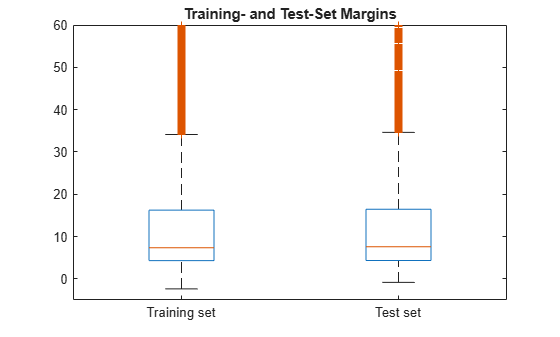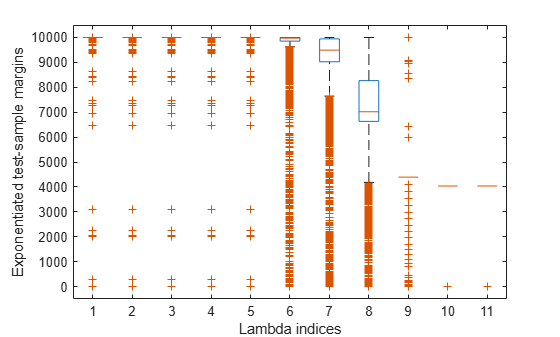margin

Class: ClassificationLinear

Classification margins for linear classification models

Description

example

m = margin(Mdl,X,Y) returns the classification margins for the binary, linear classification model Mdl using predictor data in X and corresponding class labels in Y. m contains classification margins for each regularization strength in Mdl.

example

m = margin(Mdl,X,Y,'ObservationsIn',dimension) specifies the predictor data observation dimension, either 'rows' (default) or 'columns'. For example, specify 'ObservationsIn','columns' to indicate that columns in the predictor data correspond to observations.

m = margin(Mdl,Tbl,ResponseVarName) returns the classification margins for the trained linear classifier Mdl using the predictor data in table Tbl and the class labels in Tbl.ResponseVarName.

m = margin(Mdl,Tbl,Y) returns the classification margins for the classifier Mdl using the predictor data in table Tbl and the class labels in vector Y.

Input Arguments

expand all

Binary, linear classification model, specified as a ClassificationLinear model object. You can create a ClassificationLinear model object using fitclinear.

Predictor data, specified as an n-by-p full or sparse matrix. This orientation of X indicates that rows correspond to individual observations, and columns correspond to individual predictor variables.

Note

If you orient your predictor matrix so that observations correspond to columns and specify 'ObservationsIn','columns', then you might experience a significant reduction in computation time.

The length of Y and the number of observations in X must be equal.

Data Types: single | double

Class labels, specified as a categorical, character, or string array; logical or numeric vector; or cell array of character vectors.

• The data type of Y must be the same as the data type of Mdl.ClassNames. (The software treats string arrays as cell arrays of character vectors.)

• The distinct classes in Y must be a subset of Mdl.ClassNames.

• If Y is a character array, then each element must correspond to one row of the array.

• The length of Y must be equal to the number of observations in X or Tbl.

Data Types: categorical | char | string | logical | single | double | cell

Predictor data observation dimension, specified as 'columns' or 'rows'.

Note

If you orient your predictor matrix so that observations correspond to columns and specify 'ObservationsIn','columns', then you might experience a significant reduction in optimization execution time. You cannot specify 'ObservationsIn','columns' for predictor data in a table.

Sample data used to train the model, specified as a table. Each row of Tbl corresponds to one observation, and each column corresponds to one predictor variable. Optionally, Tbl can contain additional columns for the response variable and observation weights. Tbl must contain all the predictors used to train Mdl. Multicolumn variables and cell arrays other than cell arrays of character vectors are not allowed.

If Tbl contains the response variable used to train Mdl, then you do not need to specify ResponseVarName or Y.

If you train Mdl using sample data contained in a table, then the input data for margin must also be in a table.

Response variable name, specified as the name of a variable in Tbl. If Tbl contains the response variable used to train Mdl, then you do not need to specify ResponseVarName.

If you specify ResponseVarName, then you must specify it as a character vector or string scalar. For example, if the response variable is stored as Tbl.Y, then specify ResponseVarName as 'Y'. Otherwise, the software treats all columns of Tbl, including Tbl.Y, as predictors.

The response variable must be a categorical, character, or string array; a logical or numeric vector; or a cell array of character vectors. If the response variable is a character array, then each element must correspond to one row of the array.

Data Types: char | string

Output Arguments

expand all

Classification margins, returned as a numeric column vector or matrix.

m is n-by-L, where n is the number of observations in X and L is the number of regularization strengths in Mdl (that is, numel(Mdl.Lambda)).

m(i,j) is the classification margin of observation i using the trained linear classification model that has regularization strength Mdl.Lambda(j).

Examples

expand all

X is a sparse matrix of predictor data, and Y is a categorical vector of class labels. There are more than two classes in the data.

The models should identify whether the word counts in a web page are from the Statistics and Machine Learning Toolbox™ documentation. So, identify the labels that correspond to the Statistics and Machine Learning Toolbox™ documentation web pages.

Ystats = Y == 'stats';

Train a binary, linear classification model that can identify whether the word counts in a documentation web page are from the Statistics and Machine Learning Toolbox™ documentation. Specify to hold out 30% of the observations. Optimize the objective function using SpaRSA.

rng(1); % For reproducibility
CVMdl = fitclinear(X,Ystats,'Solver','sparsa','Holdout',0.30);
CMdl = CVMdl.Trained{1};

CVMdl is a ClassificationPartitionedLinear model. It contains the property Trained, which is a 1-by-1 cell array holding a ClassificationLinear model that the software trained using the training set.

Extract the training and test data from the partition definition.

trainIdx = training(CVMdl.Partition);
testIdx = test(CVMdl.Partition);

Estimate the training- and test-sample margins.

mTrain = margin(CMdl,X(trainIdx,:),Ystats(trainIdx));
mTest = margin(CMdl,X(testIdx,:),Ystats(testIdx));

Because there is one regularization strength in CMdl, mTrain and mTest are column vectors with lengths equal to the number of training and test observations, respectively.

Plot both sets of margins using box plots.

figure;
boxplot([mTrain; mTest],[zeros(size(mTrain,1),1); ones(size(mTest,1),1)], ...
'Labels',{'Training set','Test set'});
h = gca;
h.YLim = [-5 60];
title 'Training- and Test-Set Margins'The distributions of the margins between the training and test sets appear similar.

One way to perform feature selection is to compare test-sample margins from multiple models. Based solely on this criterion, the classifier with the larger margins is the better classifier.

Load the NLP data set. Preprocess the data as in Estimate Test-Sample Margins.

Ystats = Y == 'stats';
X = X';
rng(1); % For reproducibility

Create a data partition which holds out 30% of the observations for testing.

Partition = cvpartition(Ystats,'Holdout',0.30);
testIdx = test(Partition); % Test-set indices
XTest = X(:,testIdx);
YTest = Ystats(testIdx);

Partition is a cvpartition object that defines the data set partition.

Randomly choose 10% of the predictor variables.

p = size(X,1); % Number of predictors
idxPart = randsample(p,ceil(0.1*p));

Train two binary, linear classification models: one that uses the all of the predictors and one that uses the random 10%. Optimize the objective function using SpaRSA, and indicate that observations correspond to columns.

CVMdl = fitclinear(X,Ystats,'CVPartition',Partition,'Solver','sparsa',...
'ObservationsIn','columns');
PCVMdl = fitclinear(X(idxPart,:),Ystats,'CVPartition',Partition,'Solver','sparsa',...
'ObservationsIn','columns');

CVMdl and PCVMdl are ClassificationPartitionedLinear models.

Extract the trained ClassificationLinear models from the cross-validated models.

CMdl = CVMdl.Trained{1};
PCMdl = PCVMdl.Trained{1};

Estimate the test sample margins for each classifier. Plot the distribution of the margins sets using box plots.

fullMargins = margin(CMdl,XTest,YTest,'ObservationsIn','columns');
partMargins = margin(PCMdl,XTest(idxPart,:),YTest,...
'ObservationsIn','columns');

figure;
boxplot([fullMargins partMargins],'Labels',...
{'All Predictors','10% of the Predictors'});
h = gca;
h.YLim = [-20 60];
title('Test-Sample Margins')The margin distribution of CMdl is situated higher than the margin distribution of PCMdl.

To determine a good lasso-penalty strength for a linear classification model that uses a logistic regression learner, compare distributions of test-sample margins.

Load the NLP data set. Preprocess the data as in Estimate Test-Sample Margins.

Ystats = Y == 'stats';
X = X';

Partition = cvpartition(Ystats,'Holdout',0.30);
testIdx = test(Partition);
XTest = X(:,testIdx);
YTest = Ystats(testIdx);

Create a set of 11 logarithmically-spaced regularization strengths from $1{0}^{-8}$ through $1{0}^{1}$.

Lambda = logspace(-8,1,11);

Train binary, linear classification models that use each of the regularization strengths. Optimize the objective function using SpaRSA. Lower the tolerance on the gradient of the objective function to 1e-8.

rng(10); % For reproducibility
CVMdl = fitclinear(X,Ystats,'ObservationsIn','columns',...
'CVPartition',Partition,'Learner','logistic','Solver','sparsa',...
CVMdl =
ClassificationPartitionedLinear
CrossValidatedModel: 'Linear'
ResponseName: 'Y'
NumObservations: 31572
KFold: 1
Partition: [1x1 cvpartition]
ClassNames: [0 1]
ScoreTransform: 'none'

Properties, Methods

Extract the trained linear classification model.

Mdl = CVMdl.Trained{1}
Mdl =
ClassificationLinear
ResponseName: 'Y'
ClassNames: [0 1]
ScoreTransform: 'logit'
Beta: [34023x11 double]
Bias: [-11.5378 -11.5378 -11.5378 -11.5378 -11.5378 ... ]
Lambda: [1.0000e-08 7.9433e-08 6.3096e-07 5.0119e-06 ... ]
Learner: 'logistic'

Properties, Methods

Mdl is a ClassificationLinear model object. Because Lambda is a sequence of regularization strengths, you can think of Mdl as 11 models, one for each regularization strength in Lambda.

Estimate the test-sample margins.

m = margin(Mdl,X(:,testIdx),Ystats(testIdx),'ObservationsIn','columns');
size(m)
ans = 1×2

9471          11

Because there are 11 regularization strengths, m has 11 columns.

Plot the test-sample margins for each regularization strength. Because logistic regression scores are in [0,1], margins are in [-1,1]. Rescale the margins to help identify the regularization strength that maximizes the margins over the grid.

figure;
boxplot(10000.^m)
ylabel('Exponentiated test-sample margins')
xlabel('Lambda indices')Several values of Lambda yield margin distributions that are compacted near $1000{0}^{1}$. Higher values of lambda lead to predictor variable sparsity, which is a good quality of a classifier.

Choose the regularization strength that occurs just before the centers of the margin distributions start decreasing.

LambdaFinal = Lambda(5);

Train a linear classification model using the entire data set and specify the desired regularization strength.

MdlFinal = fitclinear(X,Ystats,'ObservationsIn','columns',...
'Learner','logistic','Solver','sparsa','Regularization','lasso',...
'Lambda',LambdaFinal);

To estimate labels for new observations, pass MdlFinal and the new data to predict.

expand all